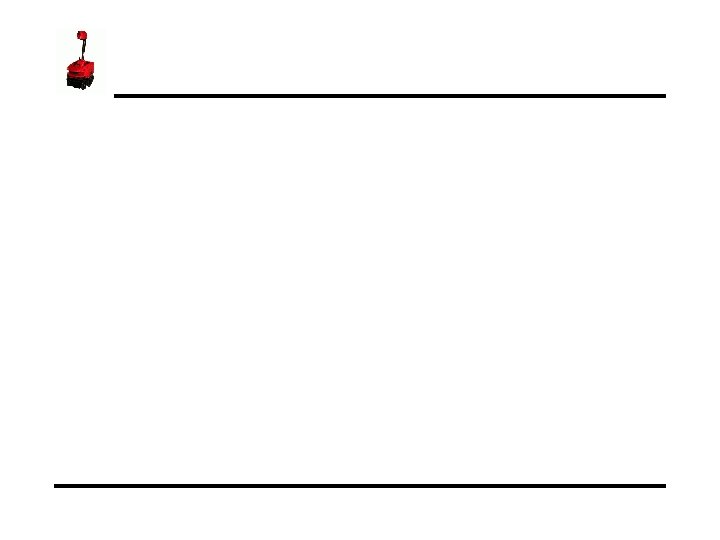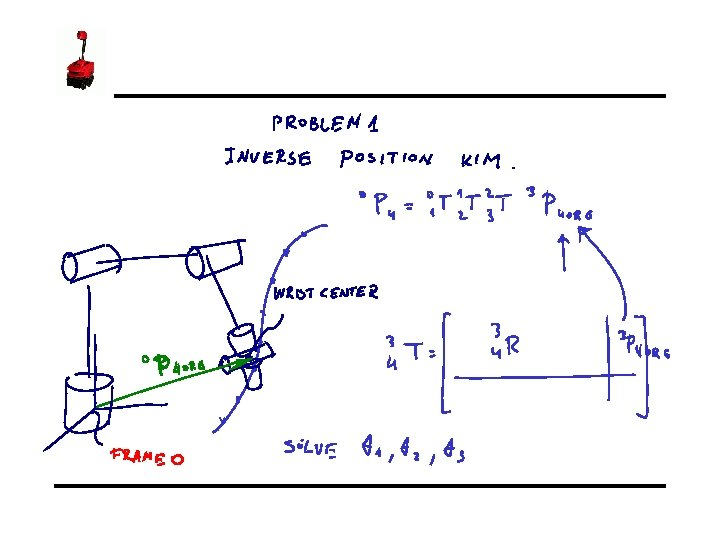# Jacobian Velocities and Static Forces 14 Instructor Jacob

• Slides: 52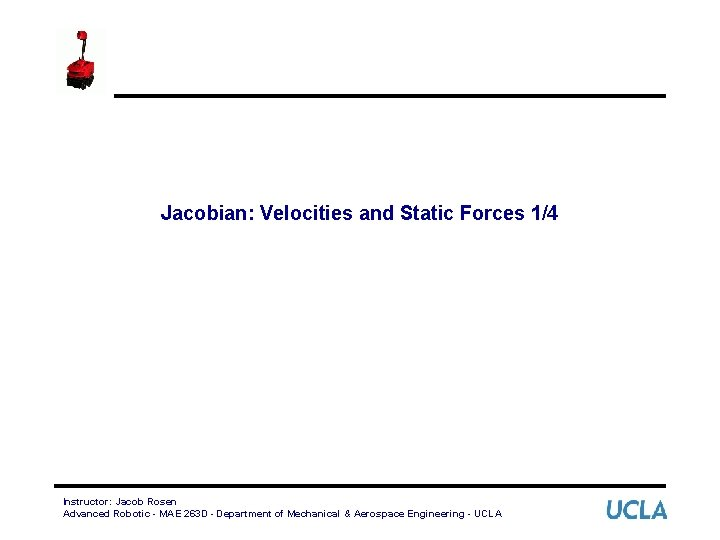Jacobian: Velocities and Static Forces 1/4 Instructor: Jacob Rosen Advanced Robotic - MAE 263 D - Department of Mechanical & Aerospace Engineering - UCLA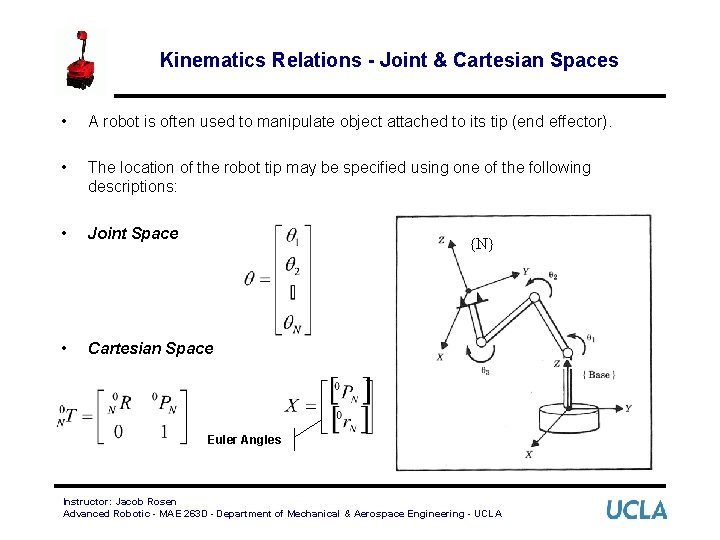Kinematics Relations - Joint & Cartesian Spaces • A robot is often used to manipulate object attached to its tip (end effector). • The location of the robot tip may be specified using one of the following descriptions: • Joint Space • Cartesian Space {N} Euler Angles Instructor: Jacob Rosen Advanced Robotic - MAE 263 D - Department of Mechanical & Aerospace Engineering - UCLAKinematics Relations - Forward & Inverse • The robot kinematic equations relate the two description of the robot tip location Tip Location in Joint Space Tip Location in Cartesian Space Instructor: Jacob Rosen Advanced Robotic - MAE 263 D - Department of Mechanical & Aerospace Engineering - UCLA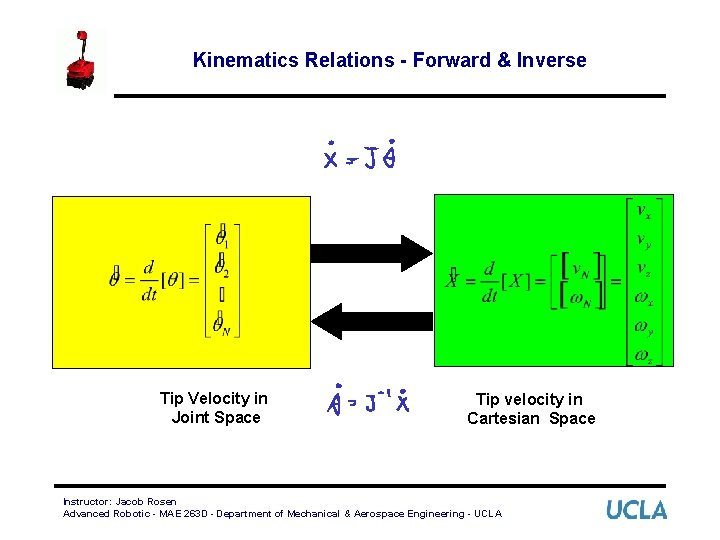Kinematics Relations - Forward & Inverse Tip Velocity in Joint Space Tip velocity in Cartesian Space Instructor: Jacob Rosen Advanced Robotic - MAE 263 D - Department of Mechanical & Aerospace Engineering - UCLA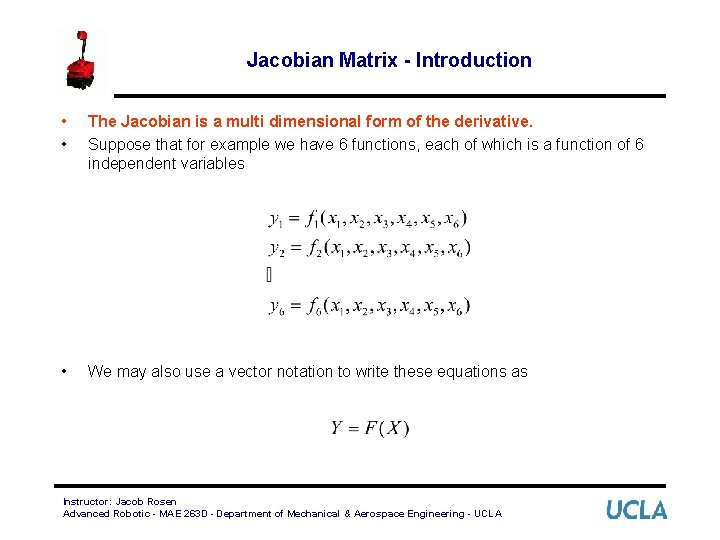Jacobian Matrix - Introduction • • The Jacobian is a multi dimensional form of the derivative. Suppose that for example we have 6 functions, each of which is a function of 6 independent variables • We may also use a vector notation to write these equations as Instructor: Jacob Rosen Advanced Robotic - MAE 263 D - Department of Mechanical & Aerospace Engineering - UCLA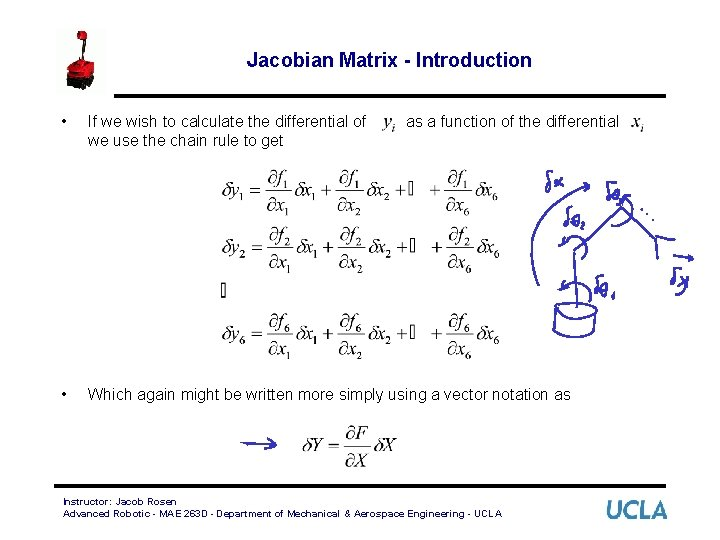Jacobian Matrix - Introduction • If we wish to calculate the differential of we use the chain rule to get • Which again might be written more simply using a vector notation as as a function of the differential Instructor: Jacob Rosen Advanced Robotic - MAE 263 D - Department of Mechanical & Aerospace Engineering - UCLA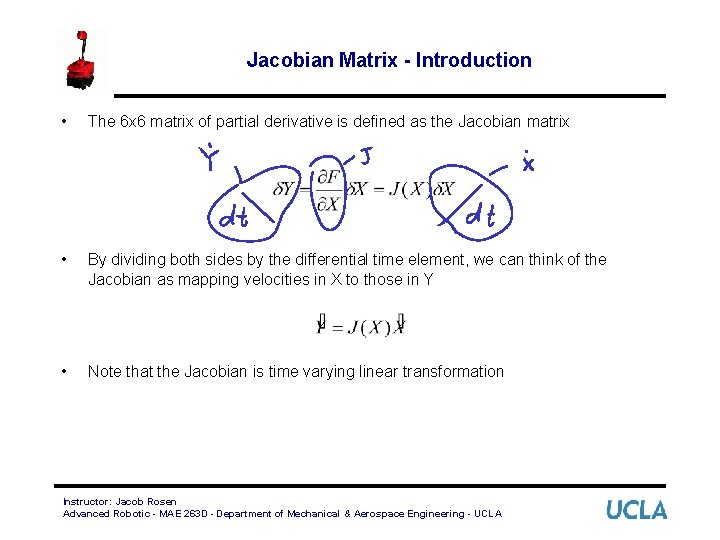Jacobian Matrix - Introduction • The 6 x 6 matrix of partial derivative is defined as the Jacobian matrix • By dividing both sides by the differential time element, we can think of the Jacobian as mapping velocities in X to those in Y • Note that the Jacobian is time varying linear transformation Instructor: Jacob Rosen Advanced Robotic - MAE 263 D - Department of Mechanical & Aerospace Engineering - UCLAJacobian Matrix - Introduction • In the field of robotics the Jacobian matrix describe the relationship between the joint angle rates ( ) and the translation and rotation velocities of the end effector ( ). This relationship is given by: Instructor: Jacob Rosen Advanced Robotic - MAE 263 D - Department of Mechanical & Aerospace Engineering - UCLAJacobian Matrix - Introduction • • This expression can be expanded to: Where: 6 x 1 6 x. N Nx 1 – is a 6 x 1 vector of the end effector linear and angular velocities – is a 6 x. N Jacobian matrix – is a Nx 1 vector of the manipulator joint velocities – is the number of joints Instructor: Jacob Rosen Advanced Robotic - MAE 263 D - Department of Mechanical & Aerospace Engineering - UCLA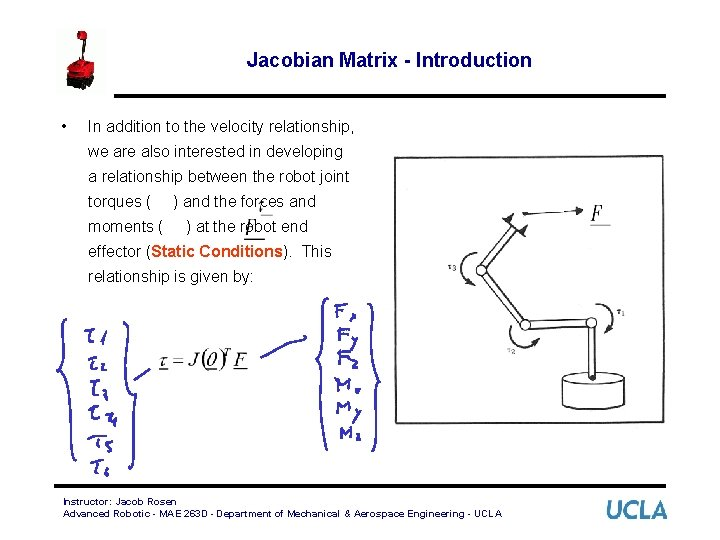Jacobian Matrix - Introduction • In addition to the velocity relationship, we are also interested in developing a relationship between the robot joint torques ( moments ( ) and the forces and ) at the robot end effector (Static Conditions). This relationship is given by: Instructor: Jacob Rosen Advanced Robotic - MAE 263 D - Department of Mechanical & Aerospace Engineering - UCLA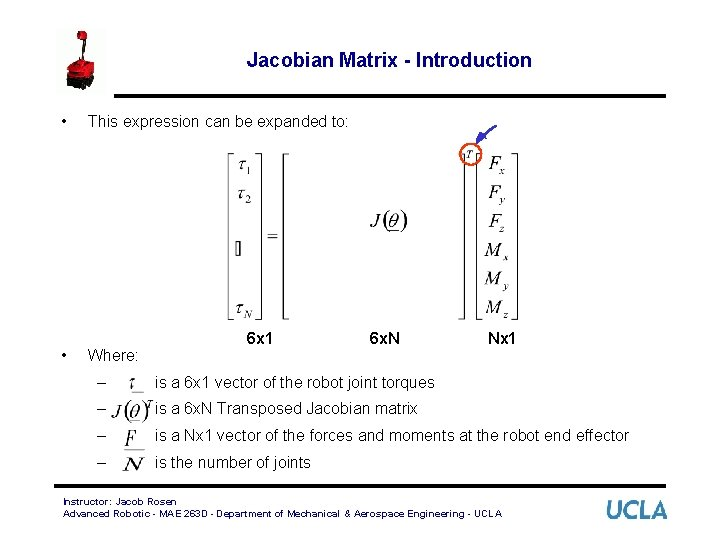Jacobian Matrix - Introduction • • This expression can be expanded to: Where: 6 x 1 6 x. N Nx 1 – is a 6 x 1 vector of the robot joint torques – is a 6 x. N Transposed Jacobian matrix – is a Nx 1 vector of the forces and moments at the robot end effector – is the number of joints Instructor: Jacob Rosen Advanced Robotic - MAE 263 D - Department of Mechanical & Aerospace Engineering - UCLAJacobian Matrix - Calculation Methods Differentiation the Forward Kinematics Eqs. Iterative Propagation (Velocities or Forces / Torques) Jacobian Matrix Instructor: Jacob Rosen Advanced Robotic - MAE 263 D - Department of Mechanical & Aerospace Engineering - UCLAJacobian Matrix by Differentiation - 1 R - 1/4 • Consider a simple planar 1 R robot • The end effector position is given by Instructor: Jacob Rosen Advanced Robotic - MAE 263 D - Department of Mechanical & Aerospace Engineering - UCLA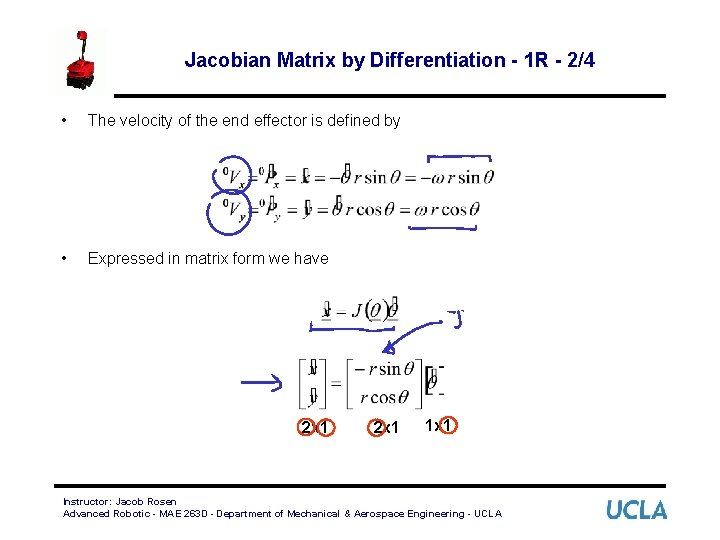Jacobian Matrix by Differentiation - 1 R - 2/4 • The velocity of the end effector is defined by • Expressed in matrix form we have 2 x 1 1 x 1 Instructor: Jacob Rosen Advanced Robotic - MAE 263 D - Department of Mechanical & Aerospace Engineering - UCLA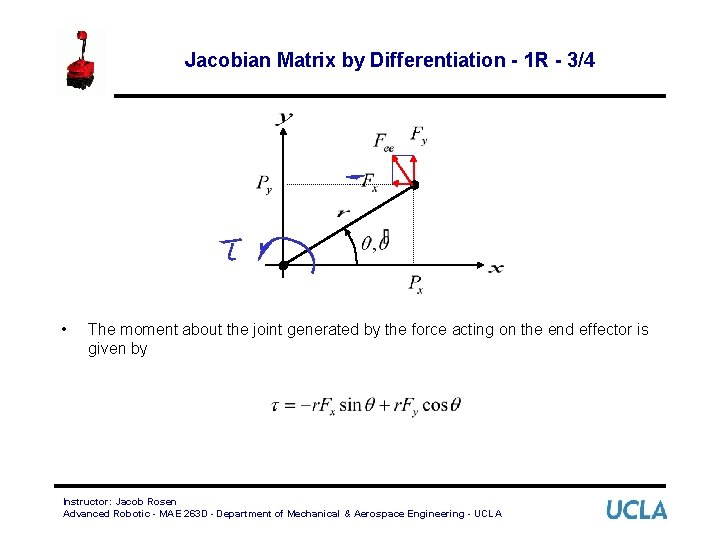Jacobian Matrix by Differentiation - 1 R - 3/4 • The moment about the joint generated by the force acting on the end effector is given by Instructor: Jacob Rosen Advanced Robotic - MAE 263 D - Department of Mechanical & Aerospace Engineering - UCLAJacobian Matrix by Differentiation - 1 R - 4/4 • Expressed in matrix form we have 1 x 1 1 x 2 2 x 1 Instructor: Jacob Rosen Advanced Robotic - MAE 263 D - Department of Mechanical & Aerospace Engineering - UCLAJacobian Matrix by Differanciation - 3 R - 1/4 • Consider the following 3 DOF Planar manipulator Instructor: Jacob Rosen Advanced Robotic - MAE 263 D - Department of Mechanical & Aerospace Engineering - UCLAJacobian Matrix by Differanciation - 3 R - 2/4 • Problem: Compute the Jacobian matrix that describes the relationship • Solution: • The end effector position and orientation is defined in the base frame by Instructor: Jacob Rosen Advanced Robotic - MAE 263 D - Department of Mechanical & Aerospace Engineering - UCLAJacobian Matrix by Differanciation - 3 R - 3/4 • The forward kinematics gives us relationship of the end effector to the joint angles: • Differentiating the three expressions gives Instructor: Jacob Rosen Advanced Robotic - MAE 263 D - Department of Mechanical & Aerospace Engineering - UCLA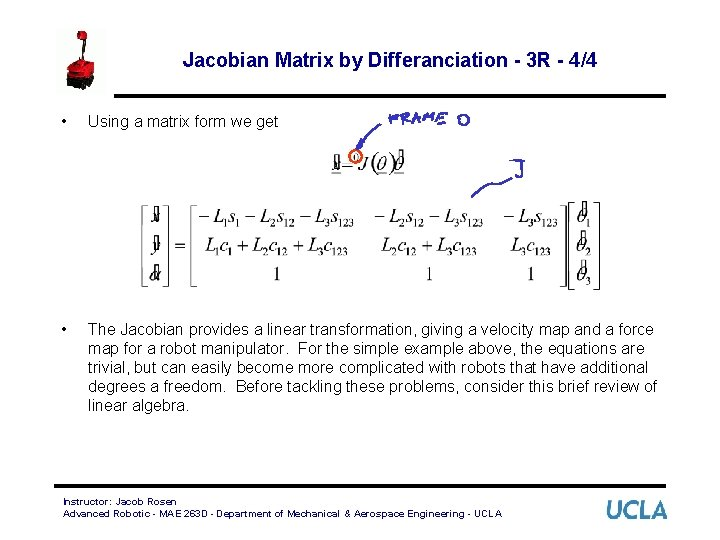Jacobian Matrix by Differanciation - 3 R - 4/4 • Using a matrix form we get • The Jacobian provides a linear transformation, giving a velocity map and a force map for a robot manipulator. For the simple example above, the equations are trivial, but can easily become more complicated with robots that have additional degrees a freedom. Before tackling these problems, consider this brief review of linear algebra. Instructor: Jacob Rosen Advanced Robotic - MAE 263 D - Department of Mechanical & Aerospace Engineering - UCLA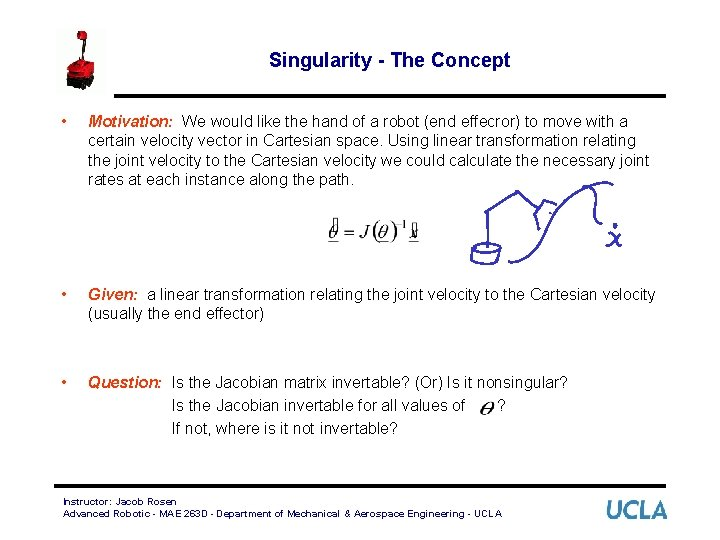Singularity - The Concept • Motivation: We would like the hand of a robot (end effecror) to move with a certain velocity vector in Cartesian space. Using linear transformation relating the joint velocity to the Cartesian velocity we could calculate the necessary joint rates at each instance along the path. • Given: a linear transformation relating the joint velocity to the Cartesian velocity (usually the end effector) • Question: Is the Jacobian matrix invertable? (Or) Is it nonsingular? Is the Jacobian invertable for all values of ? If not, where is it not invertable? Instructor: Jacob Rosen Advanced Robotic - MAE 263 D - Department of Mechanical & Aerospace Engineering - UCLASingularity - The Concept • Answer (Conceptual): Most manipulator have values of where the Jacobian becomes singular. Such locations are called singularities of the mechanism or singularities for short Singularities of the mechanism Workspace boundary singularities - Stretched out - Folded back End Effector Workspace Boundary Workspace interior Singularities - Two or more joints are lining up Instructor: Jacob Rosen Advanced Robotic - MAE 263 D - Department of Mechanical & Aerospace Engineering - UCLA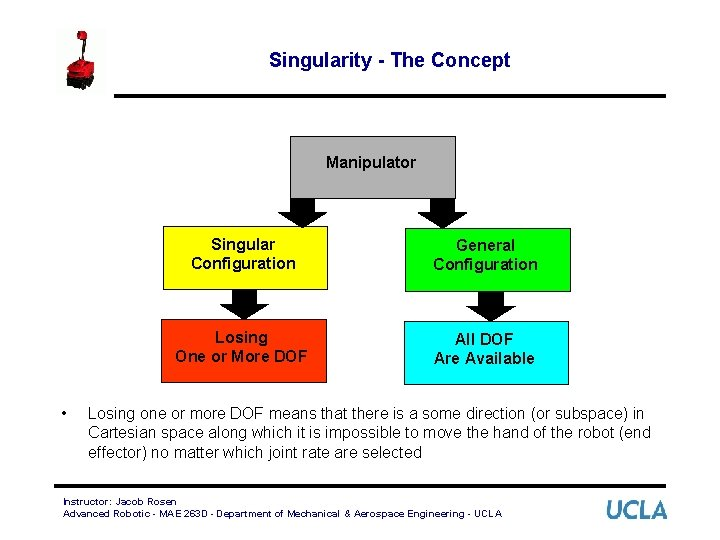Singularity - The Concept Manipulator • Singular Configuration General Configuration Losing One or More DOF All DOF Are Available Losing one or more DOF means that there is a some direction (or subspace) in Cartesian space along which it is impossible to move the hand of the robot (end effector) no matter which joint rate are selected Instructor: Jacob Rosen Advanced Robotic - MAE 263 D - Department of Mechanical & Aerospace Engineering - UCLASingularity – Physical Interpretation - Examples Instructor: Jacob Rosen Advanced Robotic - MAE 263 D - Department of Mechanical & Aerospace Engineering - UCLABrief Linear Algebra Review - 1/ • Inverse of Matrix A exists if and only if the determinant of A is non-zero. Exists if and only if • If the determinant of A is equal to zero, then the matrix A is a singular matrix Singular Instructor: Jacob Rosen Advanced Robotic - MAE 263 D - Department of Mechanical & Aerospace Engineering - UCLABrief Linear Algebra Review - 2/ • The rank of the matrix A is the size of the largest squared Matrix S for which • Example 1 - • Example 2 - Instructor: Jacob Rosen Advanced Robotic - MAE 263 D - Department of Mechanical & Aerospace Engineering - UCLA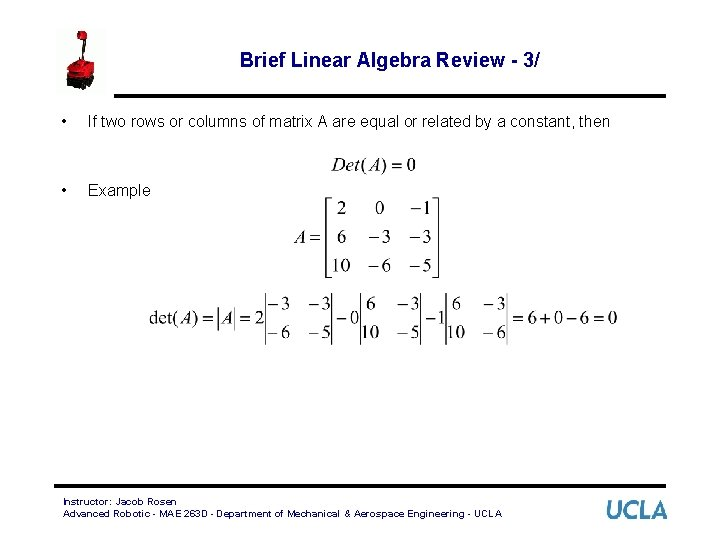Brief Linear Algebra Review - 3/ • If two rows or columns of matrix A are equal or related by a constant, then • Example Instructor: Jacob Rosen Advanced Robotic - MAE 263 D - Department of Mechanical & Aerospace Engineering - UCLABrief Linear Algebra Review - 4/ • Eigenvalues are the roots of the polynomial • If each solution to the characteristic equation corresponding Eigenvector (Eigenvalue) has a Instructor: Jacob Rosen Advanced Robotic - MAE 263 D - Department of Mechanical & Aerospace Engineering - UCLABrief Linear Algebra Review - 4/ Instructor: Jacob Rosen Advanced Robotic - MAE 263 D - Department of Mechanical & Aerospace Engineering - UCLA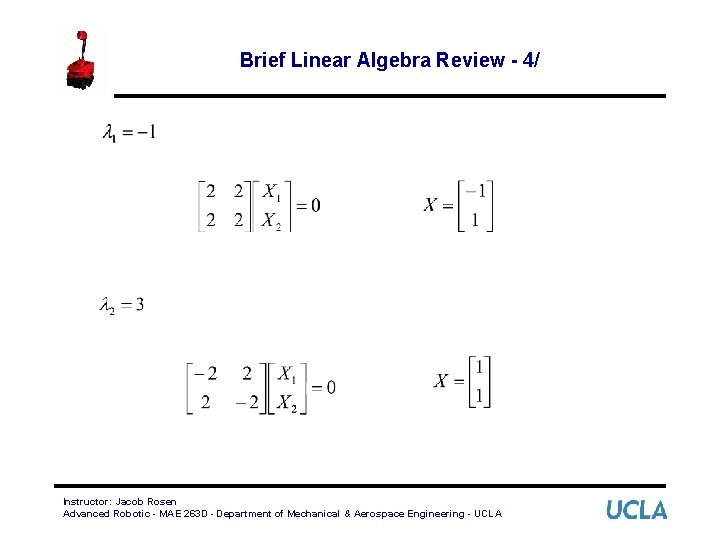Brief Linear Algebra Review - 4/ Instructor: Jacob Rosen Advanced Robotic - MAE 263 D - Department of Mechanical & Aerospace Engineering - UCLABrief Linear Algebra Review - 5/ • Any singular matrix ( ) has at least one Eigenvalue equal to zero Instructor: Jacob Rosen Advanced Robotic - MAE 263 D - Department of Mechanical & Aerospace Engineering - UCLA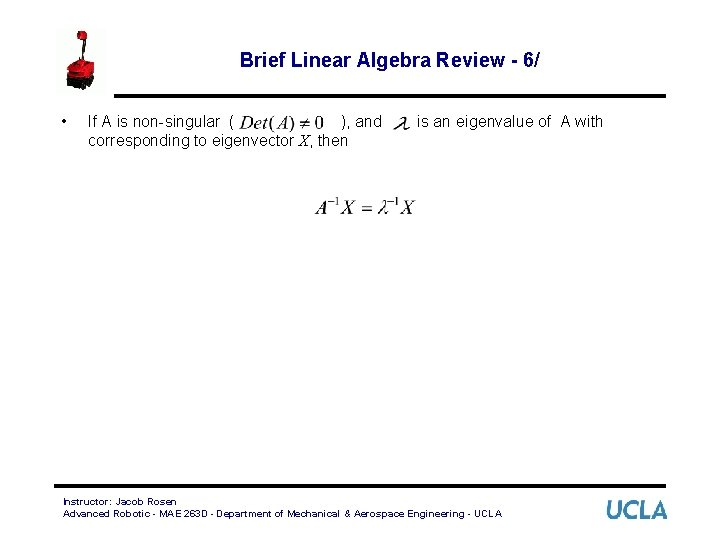Brief Linear Algebra Review - 6/ • If A is non-singular ( ), and corresponding to eigenvector X, then is an eigenvalue of A with Instructor: Jacob Rosen Advanced Robotic - MAE 263 D - Department of Mechanical & Aerospace Engineering - UCLA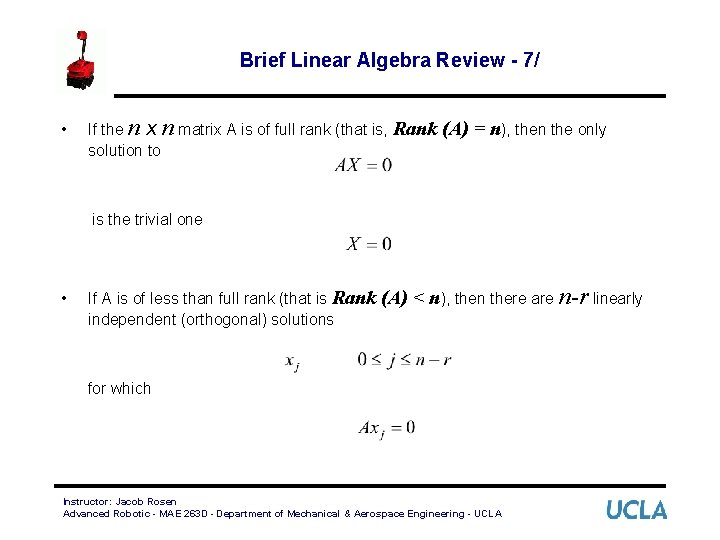Brief Linear Algebra Review - 7/ • If the n x n matrix A is of full rank (that is, Rank solution to (A) = n), then the only is the trivial one • If A is of less than full rank (that is Rank independent (orthogonal) solutions (A) < n), then there are n-r linearly for which Instructor: Jacob Rosen Advanced Robotic - MAE 263 D - Department of Mechanical & Aerospace Engineering - UCLA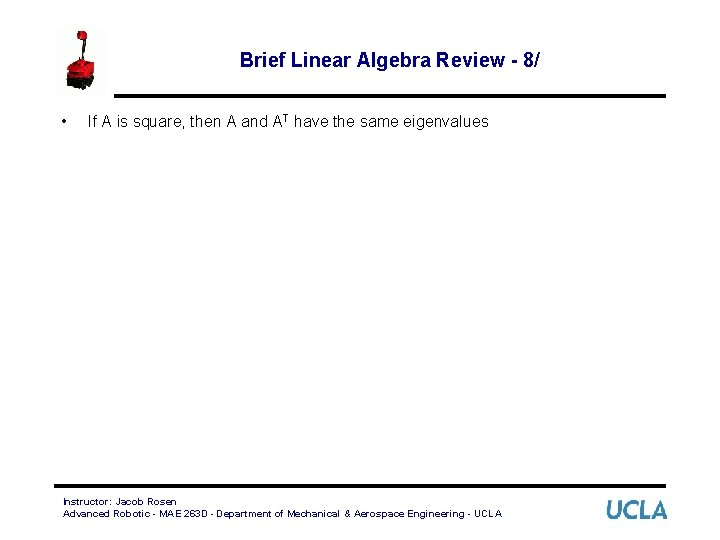Brief Linear Algebra Review - 8/ • If A is square, then A and AT have the same eigenvalues Instructor: Jacob Rosen Advanced Robotic - MAE 263 D - Department of Mechanical & Aerospace Engineering - UCLA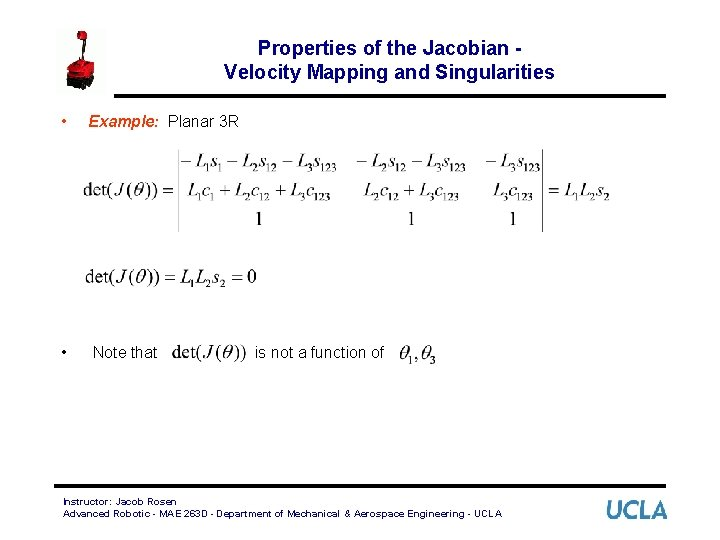Properties of the Jacobian Velocity Mapping and Singularities • Example: Planar 3 R • Note that is not a function of Instructor: Jacob Rosen Advanced Robotic - MAE 263 D - Department of Mechanical & Aerospace Engineering - UCLA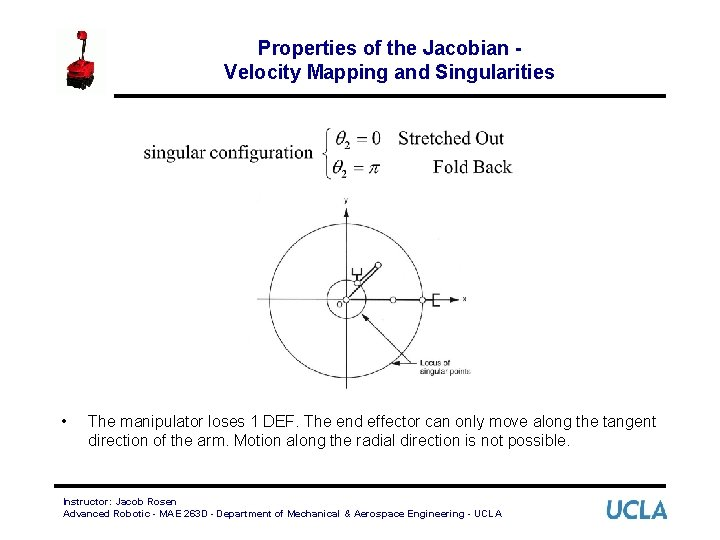Properties of the Jacobian Velocity Mapping and Singularities • The manipulator loses 1 DEF. The end effector can only move along the tangent direction of the arm. Motion along the radial direction is not possible. Instructor: Jacob Rosen Advanced Robotic - MAE 263 D - Department of Mechanical & Aerospace Engineering - UCLAProperties of the Jacobian Force Mapping and Singularities • The relationship between joint torque and effector force and moments is given by: • The rank of • At a singular configuration there exists a non trivial force • In other words, a finite force can be applied to the end effector that produces no torque at the robot’s joints. In the singular configuration, the manipulator can “lock up. ” is equals the rank of . Instructor: Jacob Rosen Advanced Robotic - MAE 263 D - Department of Mechanical & Aerospace Engineering - UCLA such thatProperties of the Jacobian Force Mapping and Singularities • Example: Planar 3 R • In this case the force acting on the end effector (relative to the {0} frame) is given by Instructor: Jacob Rosen Advanced Robotic - MAE 263 D - Department of Mechanical & Aerospace Engineering - UCLA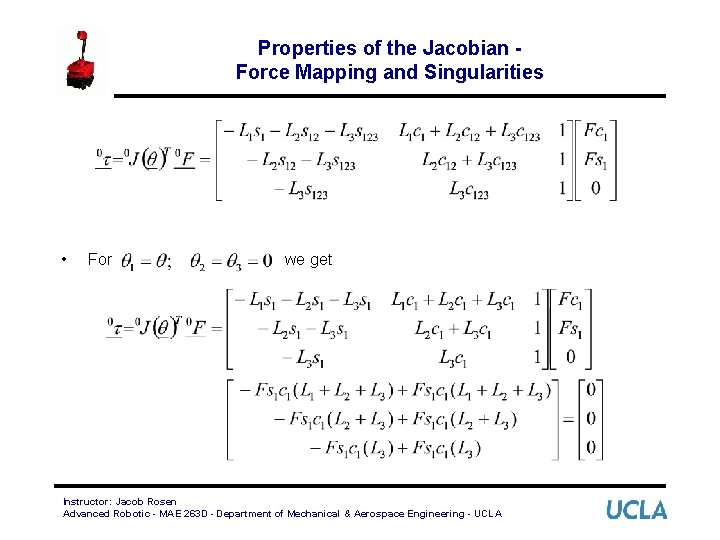Properties of the Jacobian Force Mapping and Singularities • For we get Instructor: Jacob Rosen Advanced Robotic - MAE 263 D - Department of Mechanical & Aerospace Engineering - UCLA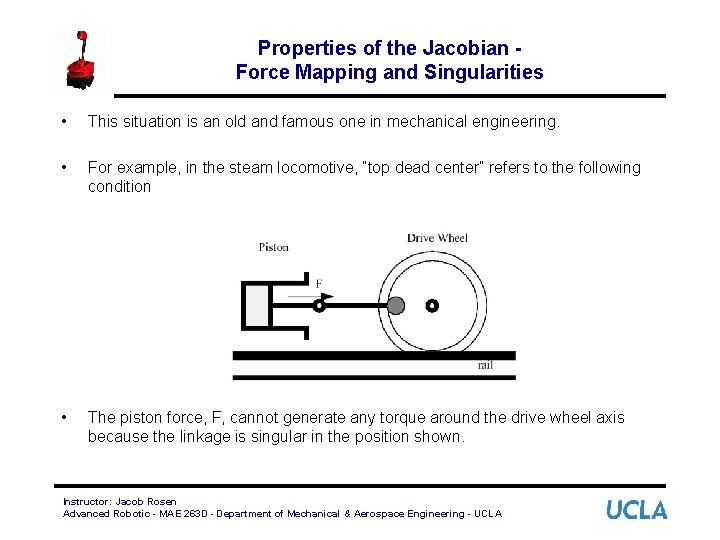Properties of the Jacobian Force Mapping and Singularities • This situation is an old and famous one in mechanical engineering. • For example, in the steam locomotive, “top dead center” refers to the following condition • The piston force, F, cannot generate any torque around the drive wheel axis because the linkage is singular in the position shown. Instructor: Jacob Rosen Advanced Robotic - MAE 263 D - Department of Mechanical & Aerospace Engineering - UCLAProperties of the Jacobian Velocity Mapping and Singularities • We have shown the relationship between joint space velocity and effector velocity, given by • It is interesting to determine the inverse of this relationship, namely Instructor: Jacob Rosen Advanced Robotic - MAE 263 D - Department of Mechanical & Aerospace Engineering - UCLAProperties of the Jacobian Velocity Mapping and Singularities • Consider the square 6 x 6 case for • If rank < 6 ( ) , then there is no solution to the inverse equation (see Brief Linear Algebra Review - 1, 7). • However, if the rank = 5, then there is at least one non-trivial solution to the forward equation (see Brief Linear Algebra Review - 7). That is, for . Instructor: Jacob Rosen Advanced Robotic - MAE 263 D - Department of Mechanical & Aerospace Engineering - UCLA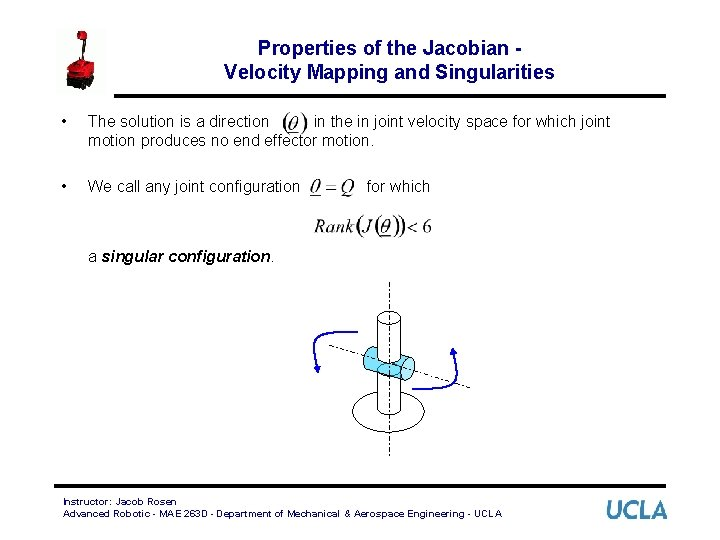Properties of the Jacobian Velocity Mapping and Singularities • The solution is a direction in the in joint velocity space for which joint motion produces no end effector motion. • We call any joint configuration for which a singular configuration. Instructor: Jacob Rosen Advanced Robotic - MAE 263 D - Department of Mechanical & Aerospace Engineering - UCLAProperties of the Jacobian Velocity Mapping and Singularities • For certain directions of end effector motion , where: – are the eigenvalues of – are the eigenvectors of • If is fully ranked (see Brief Linear Algebra Review - 6/ ), we have Instructor: Jacob Rosen Advanced Robotic - MAE 263 D - Department of Mechanical & Aerospace Engineering - UCLA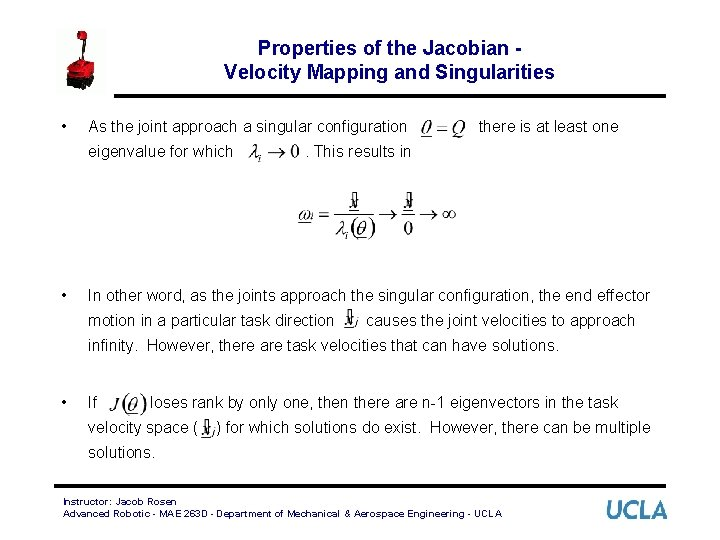Properties of the Jacobian Velocity Mapping and Singularities • As the joint approach a singular configuration eigenvalue for which • there is at least one . This results in In other word, as the joints approach the singular configuration, the end effector motion in a particular task direction causes the joint velocities to approach infinity. However, there are task velocities that can have solutions. • If loses rank by only one, then there are n-1 eigenvectors in the task velocity space ( ) for which solutions do exist. However, there can be multiple solutions. Instructor: Jacob Rosen Advanced Robotic - MAE 263 D - Department of Mechanical & Aerospace Engineering - UCLA